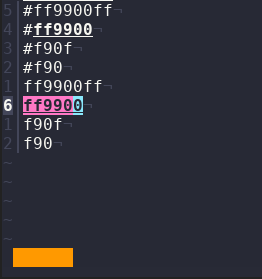# Preview hexadecimal colors in status bar

While working with CSS files it can be useful to have a preview of colors.
For example in the following stylesheet, I would like to know what this warning background looks like:

``````.warning {
background-color: #f90;
}
``````

The following kakoune command displays a block in the status bar, colored with the value of the main selection.

It can handle the following rgba formats:

• `#ff9900ff`
• `#ff9900`
• `#f90f`
• `#f90`
• `ff9900ff`
• `ff9900`
• `f90f`
• `f90`
``````define-command show-color -docstring 'show main selection color in status bar' %{
evaluate-commands %sh{
awk_script='{
if ((x=index(\$1,"#")) > 0)
\$1 = substr(\$1, x+1)
if (length(\$1) == 8)
\$1 = substr(\$1, 1, 6)
if (length(\$1) == 4)
\$1 = substr(\$1, 1, 3)
if (length(\$1) == 3) {
r = substr(\$1, 1, 1)
g = substr(\$1, 2, 1)
b = substr(\$1, 3, 1)
\$1 = r r g g b b
}
print "evaluate-commands -client " client " echo -markup {rgb:" \$1 "} ██████"
}'
printf %s\\n "\$kak_selection" | awk -v client="\$kak_client" "\$awk_script" | kak -p "\$kak_session"
}
}
``````2 Likes